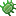# incr

The incr tag accepts as its first parameter a variable and increases the value contained within the variable by the value contained within the second optional parameter. If the second parameter is not provided, a value of 1 is assumed.

``````<cms:set my_value='11' />
<cms:show my_value />
``````

In the snippet above, value of variable my_value is outputted as 11.

``````<cms:incr my_value '2' />
<cms:show my_value />
``````

The value of variable my_value now becomes 13.

``````<cms:incr my_value />
<cms:show my_value />
``````

The value of variable my_value now becomes 14.

## Parameters

Expects a maximum of two parameters.

The first parameter has to be a variable. The second parameter is optional and its value is used to increase the value of the variable passed as the first parameter. A default value of 1 is assumed if the second parameter is not specified.

## Variables

This tag is self-closing and does not set any variables of its own.